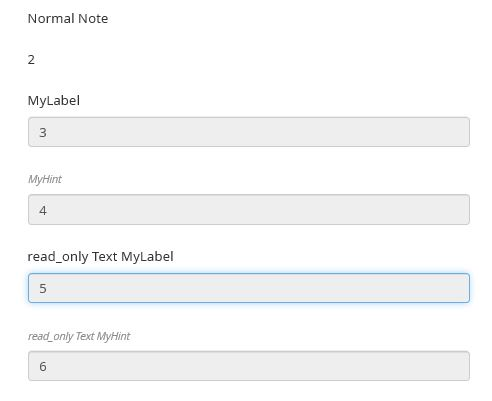# Form "date" field missing when trying to print

Hello @jborruel
Could you provide your original note code and a screenshot from the UI?
You can set the text field to read_only = true (or yes)… But it seems that there is again the problem with the KoBo print-out.
But the better solution might be first calculate variable, then \${…) reference it in the note label.

My bad, I am not expert in designing forms, and as it worked in the first place, then I assumed it posed no problem.

This is the design i have used for the tests:

type name label hint required appearan calculation default relevant \$given_name
begin_group TestDate Test Date Group false w4
select_multiple tipo_r R_type Select multiple Type false w4 horizontal TYPE1
select_multiple tipo_ R_code Select multiple ORG false w4 horizontal ORG1
date R_date Date false w2 now()
text CODE1 Text Full Calculated field false w2 concat(\${R_code},"-",format-date(\${R_date},"%Y%m%d"),"-",\${R_type},"-",\${R_code})
text CODE2 Text Calculated field - only date false w2 concat("–",format-date(\${R_date},"%Y%m%d"),"–")
note CODE3 Note Calculated field false w2 concat(\${R_code},"-",format-date(\${R_date},"%Y%m%d"),"-",\${R_type},"-",\${R_code})
note CODE4 Note Calculated field - no date false w2 concat(\${R_code},"-",\${R_type},"-",\${R_code})
note CODE5 Note Calculated field - only date false w2 concat("–",format-date(\${R_date},"%Y%m%d"),"–")
end_group

So the whole calculated field I’m using is: concat(\${R_code},"-",format-date(\${R_date},"%Y%m%d"),"-",\${R_type},"-",\${R_code})

The screenshots of this examples is in my previou posts (DateTestForm)

Thank you for your interest and support.

There are no calculated fields for R_type and R_code, it was a failure in the table copy/pasted, they are the default values… Sorry

Hello @jborruel,
If you use a Note with a label and a calculation, like you do, KoBo transforms it as a read_only text field. You can see the difference in the UI in preview.
There seems to be bug in KoBo print probably. It doesn’t show the calculated value, if a note or a text field is read_only (and has a calculation) But it seems to works ok with integer and date type.
Here are examples to illustrate:
Note_01.xlsx (10.2 KB) .
and the screenshot (Preview)and the print-out

A reported the print problem now: Print form loses calculated value for note and read_only text type